#### Variance Ratios and Standard Error Ratios

PROC SURVEYPHREG provides options to compute different variance ratios and standard error ratios.

If you specify the VARRATIO=MODEL option, then the procedure computes the variance ratio of the estimated regression parameter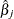as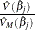, where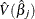, the estimated variance of, uses the complete design information and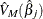is the jth diagonal element of the observed information matrix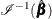.

If you specify the VARRATIO=IND option, then the procedure computes the variance ratio of the estimated regression parameteras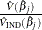, where, the estimated variance of, uses the complete design information and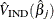is the jth diagonal element of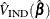.is the sandwich variance estimator, which ignores the strata and the clusters and is computed as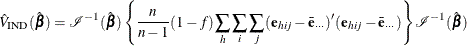where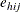are the weighted score residuals, f is the overall sampling fraction, and n is the number of observation units. The three sums are over the observation units (j) across the PSUs (i) and the strata (h).

For Taylor series variance estimation, PROC SURVEYPHREG determines the value of f, the overall sampling fraction, based on the RATE= or TOTAL= option. If you do not specify either of these options, PROC SURVEYPHREG assumes that the value of f is negligible and does not use a finite population correction in the analysis. If you specify RATE=value, PROC SURVEYPHREG uses value as the overall sampling fraction f. If you specify TOTAL=value, PROC SURVEYPHREG computes f as the ratio of the number of PSUs in the sample to the specified total.

If you specify stratum sampling rates by using the RATE=SAS-data-set option, then PROC SURVEYPHREG computes stratum totals based on these stratum sampling rates and the number of sample PSUs in each stratum. The procedure sums the stratum totals to form the overall total and then computes f as the ratio of the number of sample PSUs to the overall total. Alternatively, if you specify stratum totals with the TOTAL=SAS-data-set option, then PROC SURVEYPHREG sums these totals to compute the overall total. The overall sampling fraction f is then computed as the ratio of the number of sample PSUs to the overall total.

The replication methods do not use the finite population correction factor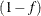in the denominator.

Standard error ratios are computed as the square root of the variance ratios.